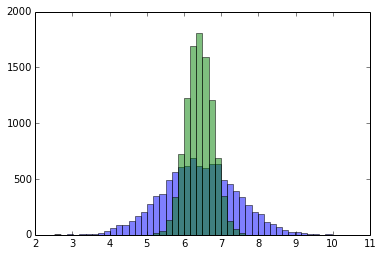# Hotelling's Experiment¶

License: BSD (C) 2014, Kyle Cranmer. Feel free to use, distribute, and modify with the above attribution.

This is an example of "Design of Experiments" - using the statistician's terminology - given in the Wikipedia article. Aimed to be used for teaching undergraduate-level physics lab / data analysis course.

The problem is to give the best measurement of the mass of 8 objects using 8 uses of a chemical balance (which has some intrensic measurement error $\sigma=1$. On a chemical balance, you can put some of your objects on the left and some on the right to measure the difference of their masses.

The code below follows the paper "On Hotelling's Weighing Problem" by Alexander M. Mood Ann. Math. Statist. Volume 17, Number 4 (1946), 377-556 which you can find here. The setup is that there is that you provide an "design matrix" that indicates where you should put each of the objects on the scale for each of the 8 weighing operations. Based on this design matrix, the code will give you the best estimate of the masses for a random experiment.

At the bottom we run 10k experiments and compare the straight-forward approach (ie. measure each object once individually) vs. Hotelling's solution.In :
%pylab inline

Populating the interactive namespace from numpy and matplotlib

In :
import numpy as np
from matplotlib import pyplot as plt

In :
class DoExperiment():
"""
This class performs a weighing experiment given true weights and a design matrix.
It follows "On Hotelling's Weighing Problem" by
Alexander M. Mood *Ann. Math. Statist.*
Volume 17, Number 4 (1946), 377-556
"""
def __init__(self, design,truthWeights,withError=True):
self._truthWeights = truthWeights
self._design = design
self._withError = withError
def get_measurement(self):
X = self._design # eq.1
truthWeights = self._truthWeights

# design is matrix where rows are weighting operations
# columns are the masses of the objects
# and entries are +1 (left), -1 (right), or 0 not on balance
measurements = dot(X,truthWeights)

#now add gaussian random error to measurements
if self._withError:
measurements += np.random.normal(0,1,len(truthWeights))

#the least squares best estimates of the true weights
aij = inv(dot(X.T,X)) #eq 2
estimates = dot(aij, dot(X,measurements)) #eq 3 & 4
return estimates

In :
#get 8 random numbers for the true weights of the objects
truthWeights = np.random.normal(10,5,8)
truthWeights

Out:
array([  6.41506648,  17.78102833,  14.06824604,   0.94515061,
13.66822659,   6.81498054,  12.80084059,  12.51520099])
In :
#the straight-forward solution to this problem is to weigh each object once.
NaiveDesign= zeros((8,8),int)
np.fill_diagonal(NaiveDesign,1)
NaiveDesign

Out:
array([[1, 0, 0, 0, 0, 0, 0, 0],
[0, 1, 0, 0, 0, 0, 0, 0],
[0, 0, 1, 0, 0, 0, 0, 0],
[0, 0, 0, 1, 0, 0, 0, 0],
[0, 0, 0, 0, 1, 0, 0, 0],
[0, 0, 0, 0, 0, 1, 0, 0],
[0, 0, 0, 0, 0, 0, 1, 0],
[0, 0, 0, 0, 0, 0, 0, 1]])
In :
#The optimal solution to this problem is based on Hadamard Matrices
from scipy.linalg import hadamard
HotellingsDesign = hadamard(8)
HotellingsDesign

Out:
array([[ 1,  1,  1,  1,  1,  1,  1,  1],
[ 1, -1,  1, -1,  1, -1,  1, -1],
[ 1,  1, -1, -1,  1,  1, -1, -1],
[ 1, -1, -1,  1,  1, -1, -1,  1],
[ 1,  1,  1,  1, -1, -1, -1, -1],
[ 1, -1,  1, -1, -1,  1, -1,  1],
[ 1,  1, -1, -1, -1, -1,  1,  1],
[ 1, -1, -1,  1, -1,  1,  1, -1]])
In :
#test that perfect experiment (with no measurement error) gives
#back the true weights
[abs(residual)<1E-5 for residual in \
(DoExperiment(HotellingsDesign,truthWeights,withError=False).get_measurement()-truthWeights)]

Out:
[True, True, True, True, True, True, True, True]
In :
#Same test for Niave Design
[abs(residual)<1E-5 for residual in \
(DoExperiment(NaiveDesign,truthWeights,withError=False).get_measurement()-truthWeights)]

Out:
[True, True, True, True, True, True, True, True]
In :
#Ok, now run 10k weighing experiments
#without loss of generality compare measurement of first object
nBins = 50
data_standard = []
data_hotelling = []
for i in range(10000):
data_standard.append(DoExperiment(NaiveDesign,truthWeights).get_measurement())
data_hotelling.append(DoExperiment(HotellingsDesign,truthWeights).get_measurement())

print( 'standard = ', np.std(data_standard), 'vs hotelling = ', np.std(data_hotelling))
print( 'observed improvement = ', np.std(data_standard)/np.std(data_hotelling))
print( 'expected improvement = ', sqrt(8))

heights, edges, patches = plt.hist(data_standard,bins=nBins, alpha=0.5)
plt.hist(data_hotelling,edges, alpha=0.5)
plt.show()

standard =  1.00535627048 vs hotelling =  0.356687120426
observed improvement =  2.81859426065
expected improvement =  2.82842712475Nice!

you can see the IPython notebook in GitHub here:

## Comments

comments powered by Disqus